#### You may also like### Calendar Capers

Choose any three by three square of dates on a calendar page...### Have You Got It?

Can you explain the strategy for winning this game with any target?### Pair Sums

Five numbers added together in pairs produce: 0, 2, 4, 4, 6, 8, 9, 11, 13, 15 What are the five numbers?

# Pyramids

##### Age 11 to 14 Challenge Level:

The problem was essentially a simple one using addition and algebra.

The solutions are:

(i)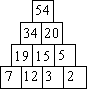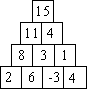(ii)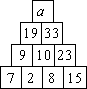This gave a = 19 + 33 = 52

(iii)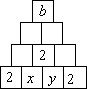All the solutions to this came from the relationship x + y = 2. The value of b was 10.

N.B. Allowing negative integers here, there are infinitely many possible values for x and y, but only one possible value of b .

(iv)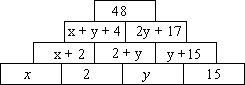The relationship for x and y is given as: x + 3 y = 27.

By choosing whole number values for y , this relationship will always give whole number values for x . Again here, allowing for negative integers , there are infinitely many possible values for x and y .